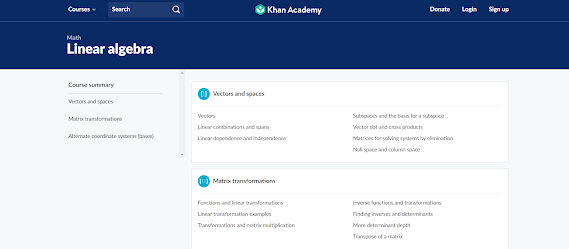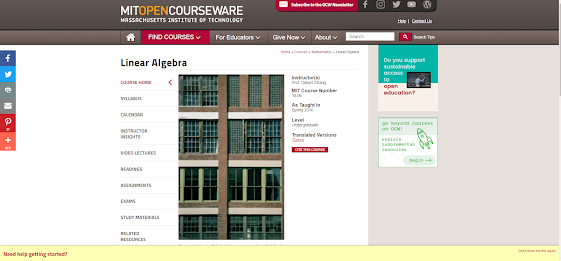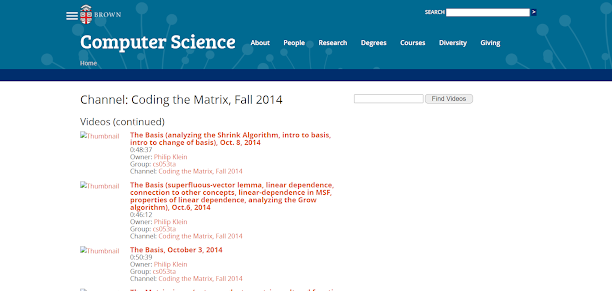# Top 5 Courses to learn Linear Algebra in Depth - Best of Lot

Hello guys, if you want to learn Linear Algebra in depth and looking for best resource slike tutorials and online courses then you have come to the right place. In the past, I have shared best Maths courses and best Statistics courses and in this article, I am going to share best online courses to learn Linear Algebra in depth . Linear algebra serves as a foundational pillar of mathematics and plays a crucial role in various fields, including computer science, physics, engineering, and data analysis. Its applications are vast, ranging from understanding complex systems to solving optimization problems. Whether you're a student, a professional looking to enhance your skills, or simply an avid learner intrigued by the beauty of mathematical concepts, finding the best linear algebra course can be a transformative step towards mastering this subject in depth.

In this article, I will guide you through the top linear algebra courses available, designed to provide comprehensive and immersive learning experiences. We will explore various online platforms and educational resources that offer exceptional courses taught by renowned instructors. Whether you prefer self-paced learning or interactive classroom settings, there is a course that caters to your preferred learning style.

Throughout our exploration, we will consider factors such as course content, teaching methodologies, practical applications, and student reviews to help you make an informed decision.
Here we are again providing a better insight into learning something which is very important and is also exciting to learn.

Now the question arises, What is linear algebra?  In simple terms, Linear algebra is a branch of mathematics, but the truth is that linear algebra is the mathematics of data. Matrices and vectors are the languages of data.

Linear algebra is about linear combinations. That is, using arithmetic on columns of numbers called  vectors and arrays of numbers called matrices, to create new columns and arrays of numbers. Linear algebra is the study of lines and planes, vector spaces, and mappings that are required for linear transforms.

.

## What are the best Linear Algebra courses you can join in 2023

Here are the 5 best online courses to learn Linear Algebra in depth:
1. Mathematics for Machine Learning: linear algebra
2. Become a linear algebra master(Udemy)
3. Learn linear algebra (khan academy)
4. Linear algebra by MIT( OCW MIT)
5. Coding the matrix by Philip Klein

Let's see each one in detail.

### 1. Mathematics for Machine Learning: linear algebra

In this course on Linear Algebra, we look at what is linear algebra is and its relation to vectors and matrices. Then we use this knowledge of matrix and vector to solve the problems of linear algebra. Here we'll be implementing some of these ideas not only in pen or paper but also on code, you'll write code blocks and encounter Jupyter notebooks in Python at the end of the course, but don't worry, these will be quite short, focussed on the concepts.

Content of course –
• There are four modules in this course named
• Introduction to linear algebra and mathematics.
• Vectors
• Matrices In linear algebra
• Matrices make linear mapping

Benefits-
• This course will also provide the certificate after completion.
• 50 video lectures for mastering the course.

### 2. Become a linear algebra master (Udemy)

This is another great course to learn about Linear Algebra online. This Udemy course is for anyone who is comfortable with the Fundamentals of Math, like arithmetic (addition, subtraction, multiplication, division) of positive and negative numbers, fractions, and decimals. This is a course full of 247 lectures with 69 quizzes.

Content of this course-

• Solving linear systems and Gauss-Jordan elimination are examples of operations on a single matrix.

• Matrix multiplication and elimination matrices are examples of operations on two matrices.

• Cauchy-Schwarz and vector triangle inequalities, as well as dot and cross product.

• Eigenvalues and Eigenvectors, encompassing eigenvalues, eigenvectors, and eigenspaces.

Benefits

• certificate on completion of course.

• Self-paced

• Full-time access

• 15-hours on-demand video.

### 3. Learn linear algebra (khan academy)

If you wanted to learn basics and want to have strong fundamentals this is a perfect course for you. The course is designed by skilled professors and industry specialists who have enough knowledge of linear algebra to turn you into a professional. You can enroll in other advanced courses after you complete  this course.

Content of the course –
• Vectors and spaces – vectors, subspaces and basis of subspaces, cross and dot product of vectors.
• Alternate coordinate system – orthogonal bases and Gram-Schmidt process, orthogonal projections.
• Matrix transformation- functions and linear transformation and inverse of it, transformation and multiplication, the question on inverses and determinantsBenefits –

• This is a free course that focuses on providing a thorough understanding of linear algebra topics.

• Learn how to define and visualize a vector, as well as some fundamental operations such as addition, subtraction, and scalar multiplication.

• Includes a variety of video lectures, quizzes, practice examinations, and real-life examples to ensure you get the most up-to-date information.

•

### 4. Linear algebra by MIT( OCW MIT)

What’s better than learning from one of the top Universities in the world MIT.

A top professor from teaching linear algebra in this course. Graphs and Networks, Systems of Differential Equations, Least Squares and Projections, and Fourier Series and the Fast Fourier Transform are the four

primary applications in the book by professor Gilbert Strang that the course highlights.

Course content-

• Elimination (pivots, multipliers, back substitution, invertibility of A, factorization into A = LU) is used to solve Ax = b for square systems.

• Ax = b (column space containing b, the rank of A, null space of A, and special solutions to Ax = 0 from row reduced R) is a complete solution.
• Solutions based on least squares (closest line by understanding projections)

• Gram-Schmidt orthogonalization (factorization into A = QR) Determinant properties (which lead to the cofactor formula and the sum over all n! permutations, as well as applications to inv(A) and volume).

• To solve difference and differential equations, eigenvalues and eigenvectors (diagonalizing A, calculating powers Ak, and matrix exponentials) are used.

• Real eigenvalues and orthogonal eigenvectors, tests for x'Ax > 0, applications)

• Symmetric matrices and positive definite matrices (real eigenvalues and orthogonal eigenvectors, tests for x'Ax > 0, applications)

• Changes in basis and linear transformations (connected to the Singular Value Decomposition - orthonormal bases that diagonalize A).Benefits-

• Excessive study materials, assignments, and 40-hours detailed videos.

• Quarterly examination to test your learnings in this course.

### 5. Coding the matrix by Philip Klein

Machine learning is based on a set of clever mathematical and logical theories and methods. This the course begins with a discussion of the limits of eigenvalue analysis before delving into the details of singular value decomposition and other matrix operations.

Content of the course-

• Law of large numbers

• The geometry of high dimensions

• Random walks in Euclidean space

• Graph partitioning

• Bayesian or belief networksBenefits

• Self-paced

• Total having 20 hours of advanced level mathematics.

#### General Questions:

Why should I learn linear algebra?
- Linear algebra is the centermost part of mathematics. In simple terms, Linear algebra allows you to comprehend geometric notions in higher dimensions, such as planes, and conduct mathematical operations on them. It may be conceived of as an arbitrary number-of-dimensional extension of algebra.

Where is Linear algebra used in real life?
- Search engine ranking, decision tree induction, software code testing in software engineering, graphics, face recognition, prediction, and other real-world applications of linear algebra are only a few examples

That's all about the best online courses to learn Linear Algebra online in 2023. So, guys what’s the wait now? you guys should go ahead and take any of the best courses for linear algebra according to your need and climb another step towards success.